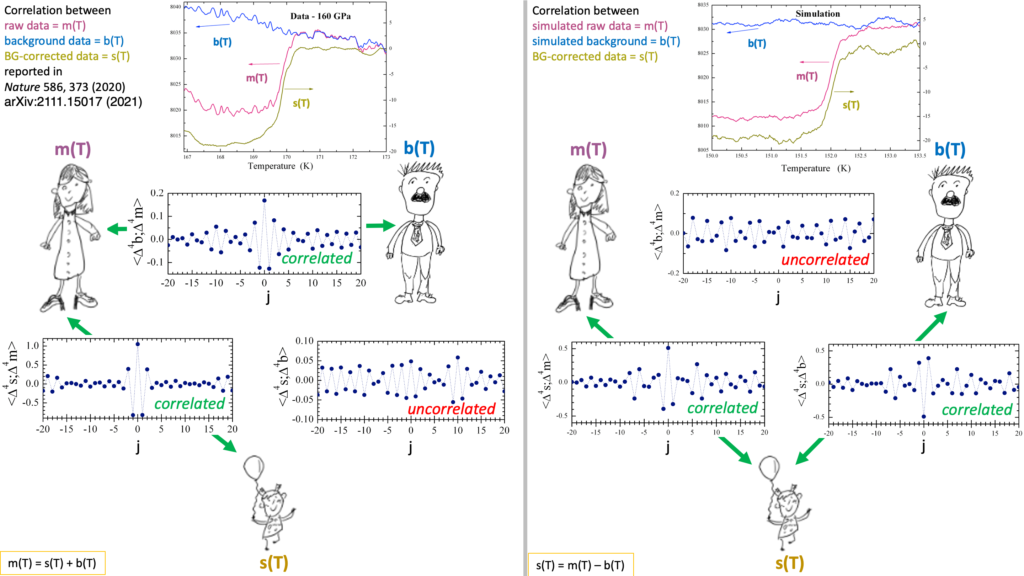# Room temperature superconductivity ?IJMPB2375001 in a nutshell: Displayed on top of the left-hand panel are the “superconducting signal” s(T) reported in Nature 586, 373 (2020), the “measured voltage” m(T) reported in arXiv:2111.15017 (2021) and the “background signal” b(T) from s(T)=m(T)-b(T). Displayed on top of the right-hand panel are a simulation of the “measured voltage” m(T), a simulation of the “background signal” b(T) and the “superconducting signal” using s(T)=m(T)-b(T). Since m(T) and b(T) are independent, the function <Δnm;Δnb>j describing the correlation between these two signals displays only random noise**. Because the background corrected signal is given by s(T)=m(T)–b(T), s(T) is necessarily correlated both with m(T) and with b(T). For <Δns;Δnm>j this is revealed as a peak and for <Δns;Δnb>j as a dip, both at j=0. The left-hand panel shows that s(T) reported in Nature 586, 373 (2020) is not compatible with a protocol where s(T) is obtained by subtracting from m(T) an independently measured signal b(T). It is compatible with the reverse protocol where b(T) and s(T) were obtained independently, causing them to be uncorrelated, and where m(T) was determined by adding b(T) to s(T). Quite obviously this clashes with the notion of m(T) representing raw data. The susceptibility data reported for the other 5 pressures (138, 166, 178, 182 and 189 GPa) exhibit the same type of correlations as those for 160 GPa.** <Δnm;Δnb>j = ΣiΔnmiΔnbi+j where i labels temperature Ti, and Δn is the nth discrete derivative, see Eq. 7 of IJMPB2375001.

Room-temperature superconductivity – or not ? Comment on Nature 586, 373 (2020) by E. Snider et al ; Dirk van der Marel & Jorge E Hirsch; International Journal of Modern Physics B 2375001 (2022), DOI:10.1142/S0217979223750012;

Incompatibility of published ac magnetic susceptibility of a room temperature superconductor with measured raw data ; J. E. Hirsch & D. van der Marel;
Matter and Radiation at Extremes 7, 048401 (2022), DOI:10.1063/5.0088429;

Room temperature superconductivity – or not ?
Flatclub seminar, University of Geneva, 29 April 2022.
Video (mp4)Slides (pptx)Slides (pdf).

Room temperature superconductivity – or not ?
Colloquium at city University of Hongkong, 7 June 2022.
Video (mp4)Slides (pptx)Slides (pdf).

“User Defined Background”. The authors of Nature 586, 373 (2020) provide various different accounts of “the making of” the susceptibility data. Pedo mellon a minno.